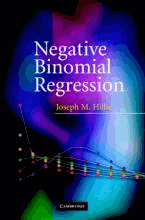# Negative Binomial Regression by Hilbe, Joseph M.

• R 400.00
Unit price per
• Save R 834.18
Tax included.

ISBN: 9780521857727

CAMBRIDGE UNIVERSITY PRESS, 27 August 2007

Hardback, 264 pages

At last - a book devoted to the negative binomial model and its many variations. Every model currently offered in commercial statistical software packages is discussed in detail - how each is derived, how each resolves a distributional problem, and numerous examples of their application. Many have never before been thoroughly examined in a text on count response models: the canonical negative binomial; the NB-P model, where the negative binomial exponent is itself parameterized; and negative binomial mixed models. As the models address violations of the distributional assumptions of the basic Poisson model, identifying and handling overdispersion is a unifying theme. For practising researchers and statisticians who need to update their knowledge of Poisson and negative binomial models, the book provides a comprehensive overview of estimating methods and algorithms used to model counts, as well as specific guidelines on modeling strategy and how each model can be analyzed to access goodness-of-fit.